# Moment of Inertia of Homogeneous Rigid Bodies | Physics – Rotational Motion

## Moment of Inertia of Homogeneous Rigid Bodies | Physics – Rotational Motion

We are giving a detailed and clear sheet on all Physics Notes that are very useful to understand the Basic Physics Concepts.

Moment of Inertia For a Thin Circular Ring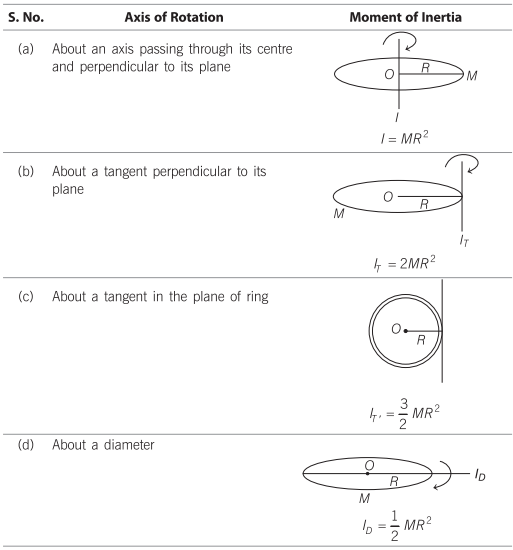Moment of Inertia For a Circular Disc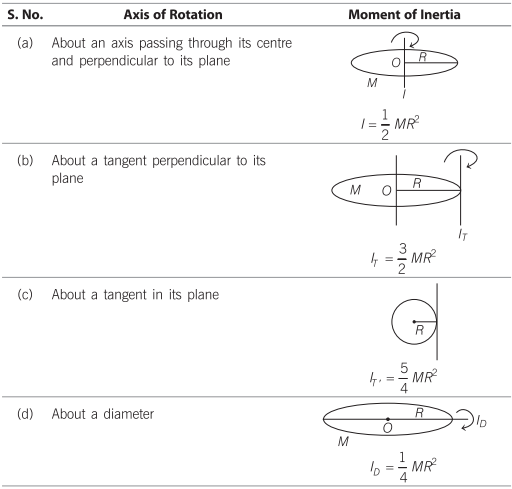Moment of Inertia For a Thin Rod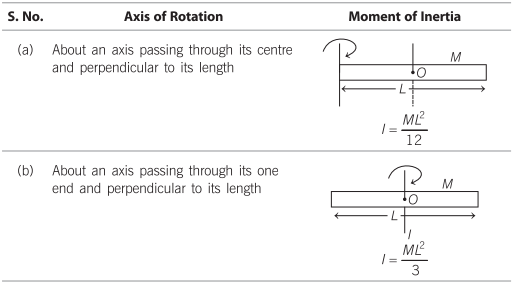Moment of Inertia For a Solid Cylinder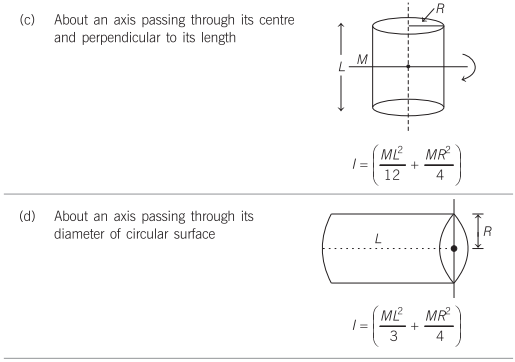Moment of Inertia For a Rectangular Plate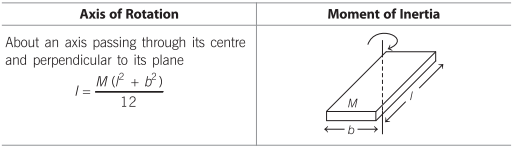Moment of Inertia For a Thin Spherical Shell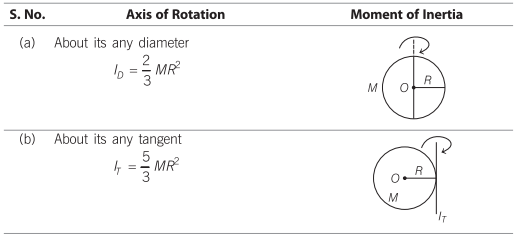Moment of Inertia For a Solid SphereRotational Motion:
In this portion, we will learn about the rotational motion of the objects. A body moves completely in rotational motion when each particle of the body moves in a circle about a single line. When a force is applied on a body about an axis it causes a rotational motion. The force applied here is called the torque. The axis of the rotation usually goes through the body. Also, learn the two theorems such as parallel axes and perpendicular theorem explained with respect to rotational motion of objects.